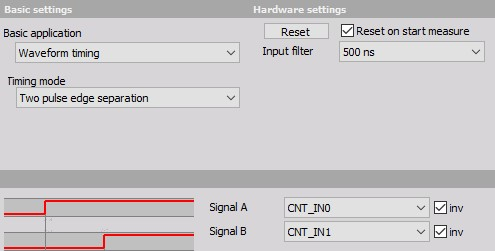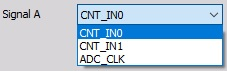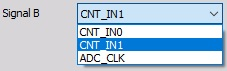# Two pulse edge separation

• Required hardware - Counter input
• Setup sample rate - At least 1 kHz

Two pulse edge separation is a special mode of counter operation. It measures the time between the rising edges of two signals. This is useful for measurement of the time between two events with a very high precision. The typical application is measured, for example, the velocity of an object passing by two sensors.

For additional help with Two pulse edge separation setup visit Dewesoft PRO training  ->Dewesoft Web page -> PRO Training.1. Select the Counter mode as ‘TwoPulseEdgeSep’.

2. Choose the Signal start and Signal stop input signal (e.g. CNT_IN0 and CNT_IN1 in above example). Both signals can be selected from the drop-down list:When both of the signals are inverted, the real trigger condition will be falling edge of the input signal, because the values are normally high when events don’t occur.

3. In the field no new value available tell the software what to do when no new value is available.

4. Set the signal Input filter to prevent glitches

We can use this value for example for speed measurements, if we are triggering (for example) from two light sensors. If we know an exact distance between them, the speed would be $v = s / t$, where $s$ is a constant and $t$ is the result of the edge separation measurement. This can be done in the math channel.